# Discrete mathematics

﻿
Discrete mathematicsGraphs like this are among the objects studied by discrete mathematics, for their interesting mathematical properties, their usefulness as models of real-world problems, and their importance in developing computer algorithms.

Discrete mathematics is the study of mathematical structures that are fundamentally discrete rather than continuous. In contrast to real numbers that have the property of varying "smoothly", the objects studied in discrete mathematics – such as integers, graphs, and statements in logic – do not vary smoothly in this way, but have distinct, separated values. Discrete mathematics therefore excludes topics in "continuous mathematics" such as calculus and analysis. Discrete objects can often be enumerated by integers. More formally, discrete mathematics has been characterized as the branch of mathematics dealing with countable sets (sets that have the same cardinality as subsets of the natural numbers, including rational numbers but not real numbers). However, there is no exact, universally agreed, definition of the term "discrete mathematics." Indeed, discrete mathematics is described less by what is included than by what is excluded: continuously varying quantities and related notions.

The set of objects studied in discrete mathematics can be finite or infinite. The term finite mathematics is sometimes applied to parts of the field of discrete mathematics that deals with finite sets, particularly those areas relevant to business.

Research in discrete mathematics increased in the latter half of the twentieth century partly due to the development of digital computers which operate in discrete steps and store data in discrete bits. Concepts and notations from discrete mathematics are useful in studying and describing objects and problems in branches of computer science, such as computer algorithms, programming languages, cryptography, automated theorem proving, and software development. Conversely, computer implementations are significant in applying ideas from discrete mathematics to real-world problems, such as in operations research.

Although the main objects of study in discrete mathematics are discrete objects, analytic methods from continuous mathematics are often employed as well.

## Grand challenges, past and present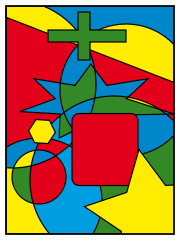Much research in graph theory was motivated by attempts to prove that all maps, like this one, could be colored with only four colors. Kenneth Appel and Wolfgang Haken finally proved this in 1976.

The history of discrete mathematics has involved a number of challenging problems which have focused attention within areas of the field. In graph theory, much research was motivated by attempts to prove the four color theorem, first stated in 1852, but not proved until 1976 (by Kenneth Appel and Wolfgang Haken, using substantial computer assistance).

In logic, the second problem on David Hilbert's list of open problems presented in 1900 was to prove that the axioms of arithmetic are consistent. Gödel's second incompleteness theorem, proved in 1931, showed that this was not possible – at least not within arithmetic itself. Hilbert's tenth problem was to determine whether a given polynomial Diophantine equation with integer coefficients has an integer solution. In 1970, Yuri Matiyasevich proved that this could not be done.

The need to break German codes in World War II led to advances in cryptography and theoretical computer science, with the first programmable digital electronic computer being developed at England's Bletchley Park. At the same time, military requirements motivated advances in operations research. The Cold War meant that cryptography remained important, with fundamental advances such as public-key cryptography being developed in the following decades. Operations research remained important as a tool in business and project management, with the critical path method being developed in the 1950s. The telecommunication industry has also motivated advances in discrete mathematics, particularly in graph theory and information theory. Formal verification of statements in logic has been necessary for software development of safety-critical systems, and advances in automated theorem proving have been driven by this need.

Computational geometry has been an important part of the computer graphics incorporated into modern video games and computer-aided design tools.

Several fields of discrete mathematics, particularly theoretical computer science, graph theory, and combinatorics, are important in addressing the challenging bioinformatics problems associated with understanding the tree of life.

Currently, one of the most famous open problems in theoretical computer science is the P = NP problem, which involves the relationship between the complexity classes P and NP. The Clay Mathematics Institute has offered a \$1 million US prize for the first correct proof, along with prizes for six other mathematical problems.

## Topics in discrete mathematics

### Theoretical computer science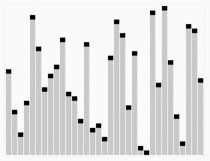Complexity studies the time taken by algorithms, such as this sorting routine.

Theoretical computer science includes areas of discrete mathematics relevant to computing. It draws heavily on graph theory and logic. Included within theoretical computer science is the study of algorithms for computing mathematical results. Computability studies what can be computed in principle, and has close ties to logic, while complexity studies the time taken by computations. Automata theory and formal language theory are closely related to computability. Petri nets and process algebras are used to model computer systems, and methods from discrete mathematics are used in analyzing VLSI electronic circuits. Computational geometry applies algorithms to geometrical problems, while computer image analysis applies them to representations of images. Theoretical computer science also includes the study of various continuous computational topics.

### Information theory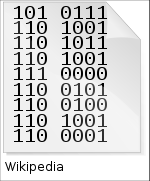The ASCII codes for the word "Wikipedia", given here in binary, provide a way of representing the word in information theory, as well as for information-processing algorithms.

Information theory involves the quantification of information. Closely related is coding theory which is used to design efficient and reliable data transmission and storage methods. Information theory also includes continuous topics such as: analog signals, analog coding, analog encryption.

### Logic

Logic is the study of the principles of valid reasoning and inference, as well as of consistency, soundness, and completeness. For example, in most systems of logic (but not in intuitionistic logic) Peirce's law (((PQ)→P)→P) is a theorem. For classical logic, it can be easily verified with a truth table. The study of mathematical proof is particularly important in logic, and has applications to automated theorem proving and formal verification of software.

Logical formulas are discrete structures, as are proofs, which form finite trees or, more generally, directed acyclic graph structures (with each inference step combining one or more premise branches to give a single conclusion). The truth values of logical formulas usually form a finite set, generally restricted to two values: true and false, but logic can also be continuous-valued, e.g., fuzzy logic. Concepts such as infinite proof trees or infinite derivation trees have also been studied, e.g. infinitary logic.

### Set theory

Set theory is the branch of mathematics that studies sets, which are collections of objects, such as {blue, white, red} or the (infinite) set of all prime numbers. Partially ordered sets and sets with other relations have applications in several areas.

In discrete mathematics, countable sets (including finite sets) are the main focus. The beginning of set theory as a branch of mathematics is usually marked by Georg Cantor's work distinguishing between different kinds of infinite set, motivated by the study of trigonometric series, and further development of the theory of infinite sets is outside the scope of discrete mathematics. Indeed, contemporary work in descriptive set theory makes extensive use of traditional continuous mathematics.

### Combinatorics

Combinatorics studies the way in which discrete structures can be combined or arranged. Enumerative combinatorics concentrates on counting the number of certain combinatorial objects - e.g. the twelvefold way provides a unified framework for counting permutations, combinations and partitions. Analytic combinatorics concerns the enumeration (i.e., determining the number) of combinatorial structures using tools from complex analysis and probability theory. In contrast with enumerative combinatorics which uses explicit combinatorial formulae and generating functions to describe the results, analytic combinatorics aims at obtaining asymptotic formulae. Design theory is a study of combinatorial designs, which are collections of subsets with certain intersection properties. Partition theory studies various enumeration and asymptotic problems related to integer partitions, and is closely related to q-series, special functions and orthogonal polynomials. Originally a part of number theory and analysis, partition theory is now considered a part of combinatorics or an independent field. Order theory is the study of partially ordered sets, both finite and infinite.

### Graph theory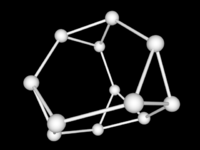Graph theory has close links to group theory. This truncated tetrahedron graph is related to the alternating group A4.

Graph theory, the study of graphs and networks, is often considered part of combinatorics, but has grown large enough and distinct enough, with its own kind of problems, to be regarded as a subject in its own right. Graphs are one of the prime objects of study in Discrete Mathematics. They are among the most ubiquitous models of both natural and human-made structures. They can model many types of relations and process dynamics in physical, biological and social systems. In computer science, they represent networks of communication, data organization, computational devices, the flow of computation, etc. In Mathematics, they are useful in Geometry and certain parts of Topology, e.g. Knot Theory. Algebraic graph theory has close links with group theory. There are also continuous graphs, however for the most part research in graph theory falls within the domain of discrete mathematics.

### Probability

Discrete probability theory deals with events that occur in countable sample spaces. For example, count observations such as the numbers of birds in flocks comprise only natural number values {0, 1, 2, ...}. On the other hand, continuous observations such as the weights of birds comprise real number values and would typically be modeled by a continuous probability distribution such as the normal. Discrete probability distributions can be used to approximate continuous ones and vice versa. For highly constrained situations such as throwing dice or experiments with decks of cards, calculating the probability of events is basically enumerative combinatorics.

### Number theory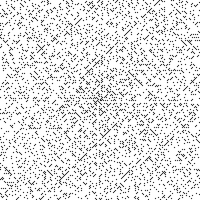The Ulam spiral of numbers, with black pixels showing prime numbers. This diagram hints at patterns in the distribution of prime numbers.

Number theory is concerned with the properties of numbers in general, particularly integers. It has applications to cryptography, cryptanalysis, and cryptology, particularly with regard to modular arithmetic, diophantine equations, linear and quadratic congruences, prime numbers and primality testing. Other discrete aspects of number theory include geometry of numbers. In analytic number theory, techniques from continuous mathematics are also used. Topics that go beyond discrete objects include transcendental numbers, diophantine approximation, p-adic analysis and function fields.

### Algebra

Algebraic structures occur as both discrete examples and continuous examples. Discrete algebras include: boolean algebra used in logic gates and programming; relational algebra used in databases; discrete and finite versions of groups, rings and fields are important in algebraic coding theory; discrete semigroups and monoids appear in the theory of formal languages.

### Calculus of finite differences, discrete calculus or discrete analysis

A function defined on an interval of the integers is usually called a sequence. A sequence could be a finite sequence from some data source or an infinite sequence from a discrete dynamical system. Such a discrete function could be defined explicitly by a list (if its domain is finite), or by a formula for its general term, or it could be given implicitly by a recurrence relation or difference equation. Difference equations are similar to a differential equations, but replace differentiation by taking the difference between adjacent terms; they can be used to approximate differential equations or (more often) studied in their own right. Many questions and methods concerning differential equations have counterparts for difference equations. For instance where there are integral transforms in harmonic analysis for studying continuous functions or analog signals, there are discrete transforms for discrete functions or digital signals. As well as the discrete metric there are more general discrete or finite metric spaces and finite topological spaces.

### Geometry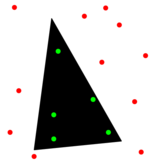Computational geometry applies computer algorithms to representations of geometrical objects.

Discrete geometry and combinatorial geometry are about combinatorial properties of discrete collections of geometrical objects. A long-standing topic in discrete geometry is tiling of the plane. Computational geometry applies algorithms to geometrical problems.

### Topology

Although topology is the field of mathematics that formalizes and generalizes the intuitive notion of "continuous deformation" of objects, it gives rise to many discrete topics; this can be attributed in part to the focus on topological invariants, which themselves usually take discrete values. See combinatorial topology, topological graph theory, topological combinatorics, computational topology, discrete topological space, finite topological space, topology (chemistry).

### Operations research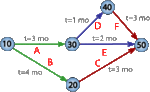PERT charts like this provide a business management technique based on graph theory.

Operations research provides techniques for solving practical problems in business and other fields — problems such as allocating resources to maximize profit, or scheduling project activities to minimize risk. Operations research techniques include linear programming and other areas of optimization, queuing theory, scheduling theory, network theory. Operations research also includes continuous topics such as continuous-time Markov process, continuous-time martingales, process optimization, and continuous and hybrid control theory.

### Game theory, decision theory, utility theory, social choice theory

 Cooperate Defect Cooperate -1, -1 -10, 0 Defect 0, -10 -5, -5 Payoff matrix for the Prisoner's dilemma, a common example in game theory. One player chooses a row, the other a column; the resulting pair gives their payoffs

Decision theory is concerned with identifying the values, uncertainties and other issues relevant in a given decision, its rationality, and the resulting optimal decision.

Utility theory is about measures of the relative economic satisfaction from, or desirability of, consumption of various goods and services.

Social choice theory is about voting. A more puzzle-based approach to voting is ballot theory.

Game theory deals with situations where success depends on the choices of others, which makes choosing the best course of action more complex. There are even continuous games, see differential game. Topics include auction theory and fair division.

### Discretization

Discretization concerns the process of transferring continuous models and equations into discrete counterparts, often for the purposes of making calculations easier by using approximations. Numerical analysis provides an important example.

### Discrete analogues of continuous mathematics

There are many concepts in continuous mathematics which have discrete versions, such as discrete calculus, discrete probability distributions, discrete Fourier transforms, discrete geometry, discrete logarithms, discrete differential geometry, discrete exterior calculus, discrete Morse theory, difference equations, discrete dynamical systems, and discrete vector measures.

In applied mathematics, discrete modelling is the discrete analogue of continuous modelling. In discrete modelling, discrete formulae are fit to data. A common method in this form of modelling is to use recurrence relations.

### Hybrid discrete and continuous mathematics

The time scale calculus is a unification of the theory of difference equations with that of differential equations, which has applications to fields requiring simultaneous modelling of discrete and continuous data.

Wikimedia Foundation. 2010.

### Look at other dictionaries:

• discrete mathematics — noun A blanket term which includes most discrete or computer science related branches of mathematics, such as graph theory and combinatorics …   Wiktionary

• Discrete Mathematics (journal) — For the area of mathematics, see Discrete mathematics. Discrete Mathematics   Abbreviated title ( …   Wikipedia

• List of basic discrete mathematics topics — Discrete mathematics, also called finite mathematics, is the study of mathematical structures that are fundamentally , in the sense of not supporting or requiring the notion of continuity. Most, if not all, of the objects studied in finite… …   Wikipedia

• Outline of discrete mathematics — The following outline is presented as an overview of and topical guide to discrete mathematics: Discrete mathematics – study of mathematical structures that are fundamentally discrete rather than continuous. In contrast to real numbers that have… …   Wikipedia

• Discharging method (discrete mathematics) — The discharging method is a technique used to prove lemmas in structural graph theory. Discharging is most well known for its central role in the proof of the Four Color Theorem. The discharging method is used to prove that every graph in a… …   Wikipedia

• Discrete — in science is the opposite of continuous: something that is separate; distinct; individual. This article is about the possible uses of the word discrete . For a definition of the word discreet , see the Wiktionary entry discreet. Discrete may… …   Wikipedia

• Discrete geometry — A collection of circles and the corresponding unit disk graph Combinatorial geometry redirects here. The term combinatorial geometry is also used in the theory of matroids to refer to a simple matroid, especially in older texts. Discrete geometry …   Wikipedia

• Discrete tomography — A discrete tomography reconstruction problem for two vertical and horizontal directions (left), together with its (non unique) solution (right). The task is to color some of the white points black so that the number of black points in the rows… …   Wikipedia

• discrete — 01. The [discrete] sound difference between the vowels /ou/ and /u/ in French can be difficult for English speakers to hear. 02. The instruction manual for the software breaks down the installation process into a series of [discrete] steps. 03. A …   Grammatical examples in English

• Mathematics — Maths and Math redirect here. For other uses see Mathematics (disambiguation) and Math (disambiguation). Euclid, Greek mathematician, 3r …   Wikipedia